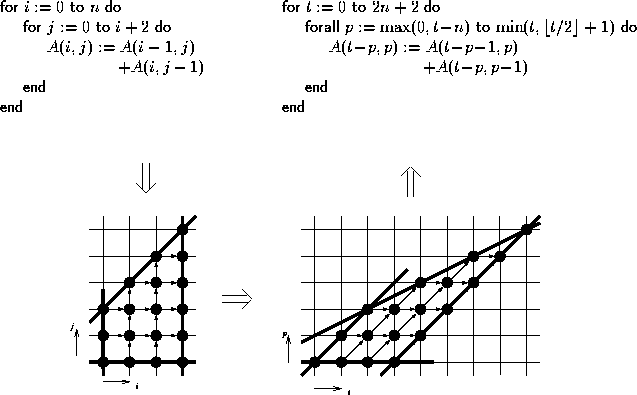Next: Extension to affine-by-statement scheduling Up: Theoretical Background Previous: Theoretical Background

## The basic polytope methodFigure 1: Parallelization in the model

Parallelization in the polytope model proceeds as follows (Figure 1).

First, one transforms d perfectly nested source loops into a d-dimensional polytope, where each loop defines the extent of the polytope in one dimension. We call this polytope the index space. Each point of the index space represents one iteration step of the loop nest. The coordinates of the point are given by the values of the loop indices at that step; the vector of these coordinates is called the index vector.

Then one applies an affine coordinate transformation, the space-time mapping, to the polytope and obtains another polytope in which some dimensions enumerate space and the others enumerate time. We call the transformed polytope the target space. The standard techniques for space-time mapping in the polytope model require uniform dependences [KMW67]. In this case, all points of the index space have identical dependence vectors which are independent of the problem size. LooPo accepts any affine, not only uniform dependences.

Finally, one translates this polytope back to a nest of target loops, where each space dimension becomes a parallel loop and each time dimension becomes a sequential loop.

Martin Griebl
Wed Apr 24 11:24:00 MET DST 1996